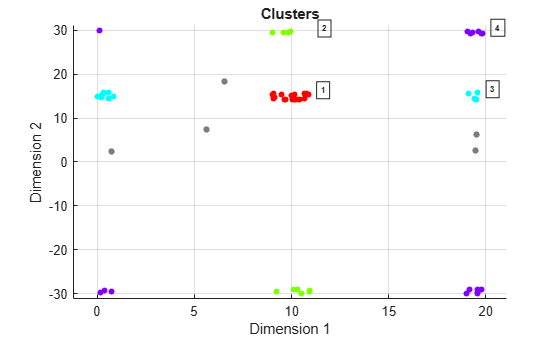# clusterDBSCAN.plot

## Syntax

``fh = plot(clusterer,X,idx)``
``fh = plot(___,'Parent',ax)``
``fh = plot(___,'Title',titlestr)``

## Description

example

````fh = plot(clusterer,X,idx)` displays a plot of DBSCAN clustering results and returns a figure handle, `fh`. Inputs are the cluster object, `clusterer`, the input data matrix, `X`, and cluster indices, `idx`.```
````fh = plot(___,'Parent',ax)` also specifies the axes, `ax`, of the cluster results plot.```
````fh = plot(___,'Title',titlestr)` also specifies the title, `titlestr`, of the cluster results plot.```

## Examples

collapse all

Create detections of extended objects with measurements in range and Doppler. Assume the maximum unambiguous range is 20 m and the unambiguous Doppler span extends from $-30$ Hz to $30$ Hz. Data for this example is contained in the `dataClusterDBSCAN.mat` file. The first column of the data matrix represents range, and the second column represents Doppler.

The input data contains the following extended targets and false alarms:

• an unambiguous target located at $\left(10,15\right)$

• an ambiguous target in Doppler located at$\left(10,-30\right)$

• an ambiguous target in range located at $\left(20,15\right)$

• an ambiguous target in range and Doppler located at $\left(20,30\right)$

• 5 false alarms

Create a `clusterDBSCAN` object and specify that disambiguation is not performed by setting `EnableDisambiguation` to `false`. Solve for the cluster indices.

```load('dataClusterDBSCAN.mat'); cluster1 = clusterDBSCAN('MinNumPoints',3,'Epsilon',2, ... 'EnableDisambiguation',false); idx = cluster1(x);```

Use the `clusterDBSCAN` `plot` object function to display the clusters.

`plot(cluster1,x,idx)`The plot indicates that there are eight apparent clusters and six noise points. The '`Dimension 1'` label corresponds to range and the '`Dimension 2'` label corresponds to Doppler.

Next, create another `clusterDBSCAN` object and set `EnableDisambiguation` to `true` to specify that clustering is performed across the range and Doppler ambiguity boundaries.

```cluster2 = clusterDBSCAN('MinNumPoints',3,'Epsilon',2, ... 'EnableDisambiguation',true,'AmbiguousDimension',[1 2]);```

Perform the clustering using ambiguity limits and then plot the clustering results. The DBSCAN clustering results correctly show four clusters and five noise points. For example, the points at ranges close to zero are clustered with points near 20 m because the maximum unambiguous range is 20 m.

```amblims = [0 maxRange; minDoppler maxDoppler]; idx = cluster2(x,amblims); plot(cluster2,x,idx)```## Input Arguments

collapse all

Clusterer object, specified as a `clusterDBSCAN` object.

Input data, specified as a real-valued N-by-P matrix. The N rows correspond to points in a P-dimensional feature space. The P columns contain the values of the features over which clustering takes place. For example, a two-column input can contain Cartesian coordinates x and y, or range and Doppler.

Data Types: `double`

Cluster indices, specified as an N-by-1 integer-valued column vector. Cluster indices represent the clustering results of the DBSCAN algorithm contained in the first output argument of `clusterDBSCAN`. `idx` values start at one and are consecutively numbered. The plot object function labels each cluster with the cluster index. A value of –1 in `idx` indicates a DBSCAN noise point. Noise points are not labeled.

Data Types: `double`

Axes of plot, specified as an `Axes` object handle.

Data Types: `double`

Plot title, specified as a character vector or string.

Example: `'Range-Doppler Clusters'`

Data Types: `char` | `string`

## Output Arguments

collapse all

Figure handle of plot, returned as a positive scalar.

## Version History

Introduced in R2021a